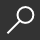menu

## A First Course in Linear Algebra by Robert A. Beezer

Overview -
A First Course in Linear Algebra is an introduction to the basic concepts of linear algebra, along with an introduction to the techniques of formal mathematics. It begins with systems of equations and matrix algebra before moving into the theory of abstract vector spaces, eigenvalues, linear transformations and matrix representations. It has numerous worked examples and exercises, along with precise statements of definitions and complete proofs of every theorem, making it ideal for independent study.

Read Full Product Description

local_shippingFor DeliveryIn Stock.
FREE Shipping for Club Members help

storeBuy Online Pickup At StoreNew & Used Marketplace 9 copies from \$30.29

## More About A First Course in Linear Algebra by Robert A. Beezer

### Overview

A First Course in Linear Algebra is an introduction to the basic concepts of linear algebra, along with an introduction to the techniques of formal mathematics. It begins with systems of equations and matrix algebra before moving into the theory of abstract vector spaces, eigenvalues, linear transformations and matrix representations. It has numerous worked examples and exercises, along with precise statements of definitions and complete proofs of every theorem, making it ideal for independent study.

### Details

• ISBN-13: 9780984417551
• ISBN-10: 0984417559
• Publisher: Congruent Press
• Publish Date: December 2012
• Page Count: 542
• Dimensions: 10 x 7 x 1.19 inches
• Shipping Weight: 2.53 poundsRelated Categories